# Evaluating Hypothesis Evaluating the accuracy of hypotheses is

• Slides: 20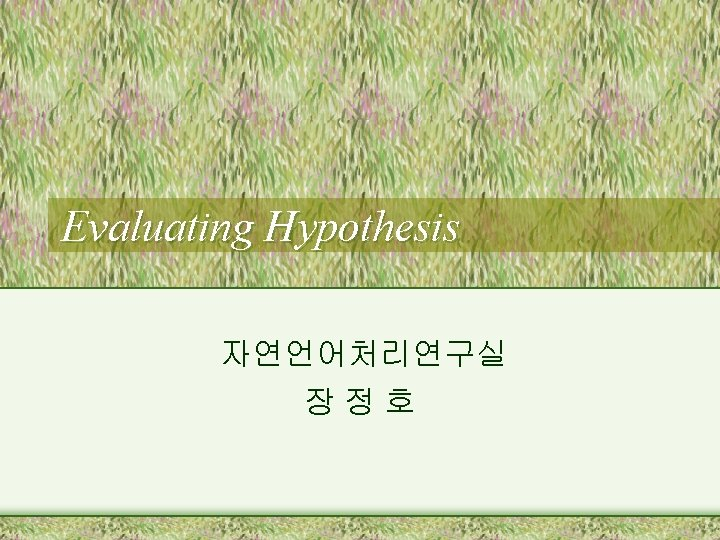Evaluating Hypothesis 자연언어처리연구실 장정호개요 • Evaluating the accuracy of hypotheses is fundamental to ML. - to decide whether to use this hypothesis - integral component of many learning system • Difficulty from limited set of data - Bias in the estimate - Variance in the estimate1. Contents • Methods for evaluating learned hypotheses • Methods for comparing the accuracy of two learning algorithms when limited set of data is available2. Estimating Hypothesis Accuracy • Two Interests 1. Given a hypothesis h and a data sample, what is the best estimate of the accuracy of h over unseen data? 2. What is probable error in accuracy estimate?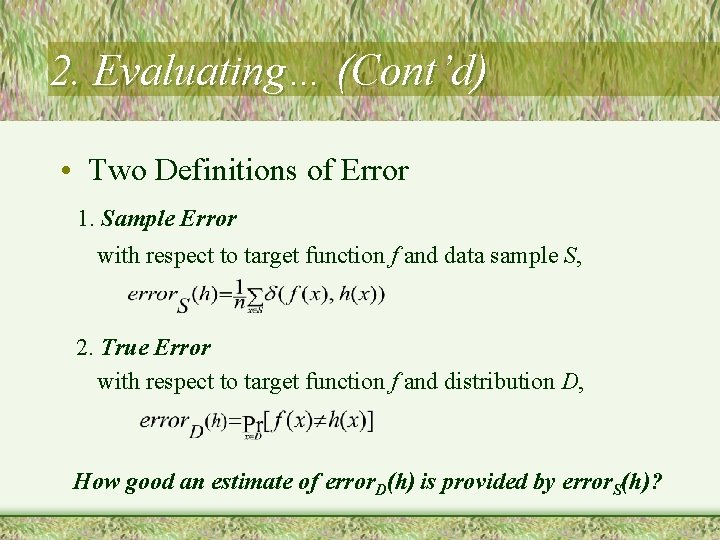2. Evaluating… (Cont’d) • Two Definitions of Error 1. Sample Error with respect to target function f and data sample S, 2. True Error with respect to target function f and distribution D, How good an estimate of error. D(h) is provided by error. S(h)?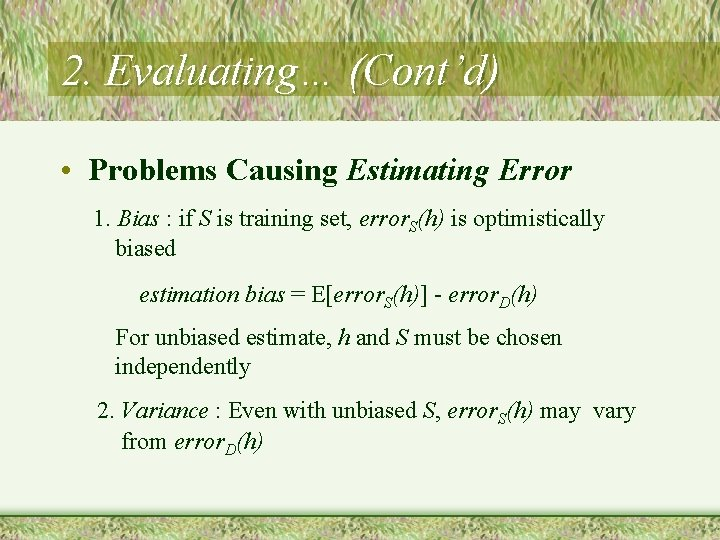2. Evaluating… (Cont’d) • Problems Causing Estimating Error 1. Bias : if S is training set, error. S(h) is optimistically biased estimation bias = E[error. S(h)] - error. D(h) For unbiased estimate, h and S must be chosen independently 2. Variance : Even with unbiased S, error. S(h) may vary from error. D(h)2. Evaluating… (Cont’d) • Estimators Experiment : 1. Choose sample S of size n according to distribution D 2. Measure error. S(h) is a random variable error. S(h) is an unbiased estimator for error. D(h) Given observed error. S(h) what can we conclude about error. D(h) ?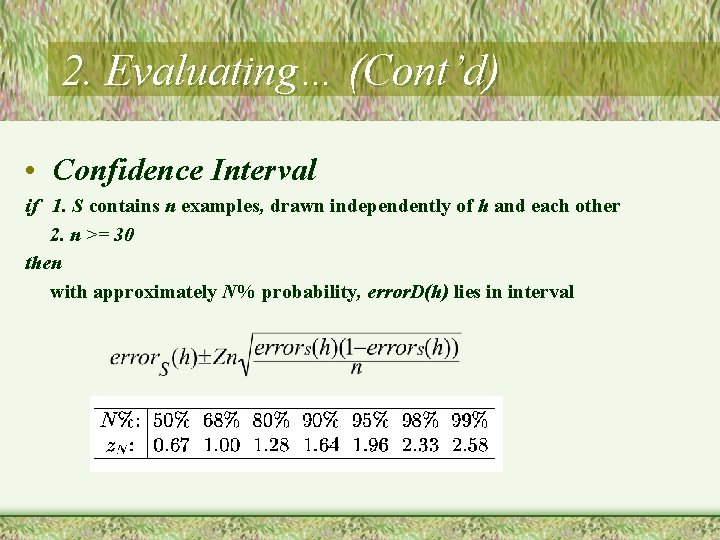2. Evaluating… (Cont’d) • Confidence Interval if 1. S contains n examples, drawn independently of h and each other 2. n >= 30 then with approximately N% probability, error. D(h) lies in interval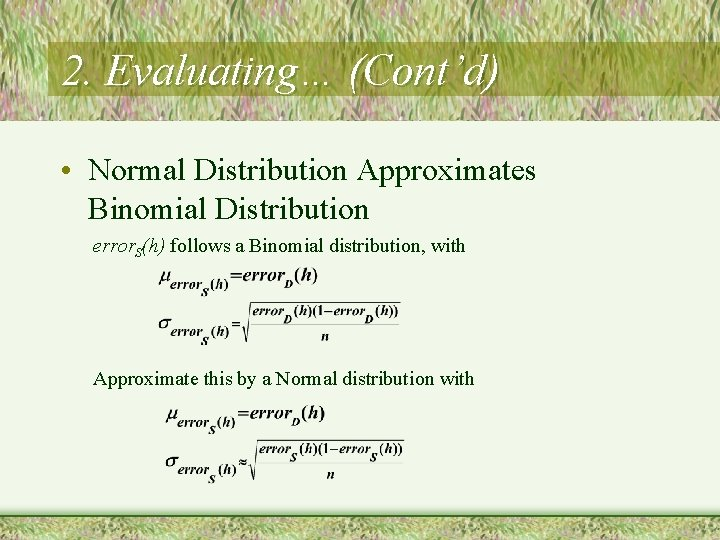2. Evaluating… (Cont’d) • Normal Distribution Approximates Binomial Distribution error. S(h) follows a Binomial distribution, with Approximate this by a Normal distribution with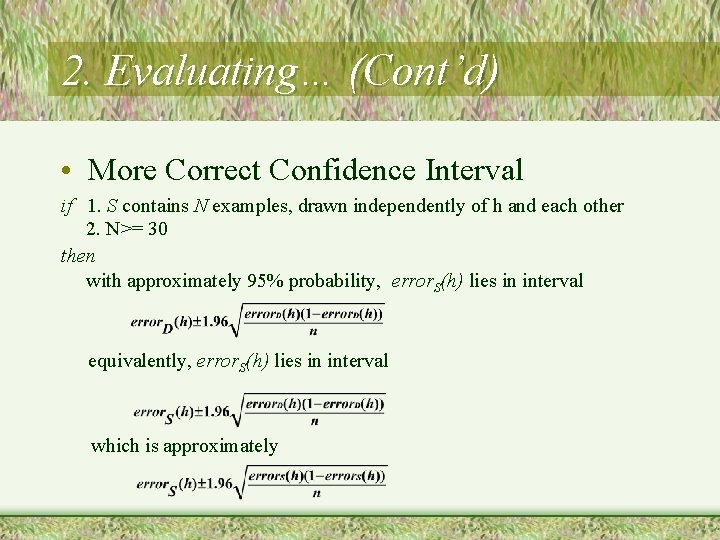2. Evaluating… (Cont’d) • More Correct Confidence Interval if 1. S contains N examples, drawn independently of h and each other 2. N>= 30 then with approximately 95% probability, error. S(h) lies in interval equivalently, error. S(h) lies in interval which is approximately2. Evaluating… (Cont’d) • Two-sided and One-sided bounds 1. Two-sided What is the probability that error. D(h) is between L and U? 2. One-sided What is the probability that error. D(h) is at most U? 100(1 -a)% confidence interval in Two-sided implies 100(1 -a/2)% in One-sided.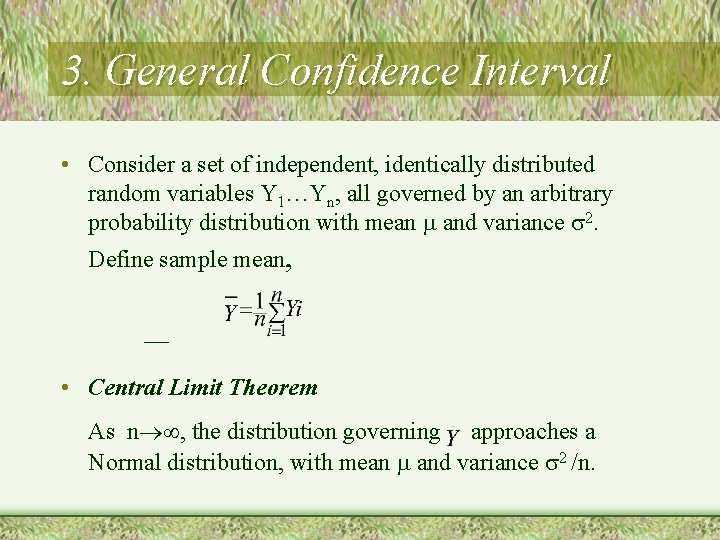3. General Confidence Interval • Consider a set of independent, identically distributed random variables Y 1…Yn, all governed by an arbitrary probability distribution with mean and variance 2. Define sample mean, • Central Limit Theorem As n , the distribution governing approaches a Normal distribution, with mean and variance 2 /n.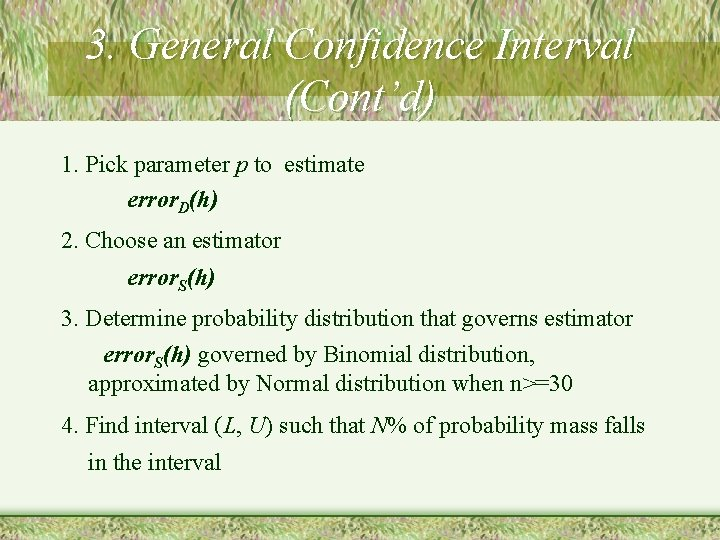3. General Confidence Interval (Cont’d) 1. Pick parameter p to estimate error. D(h) 2. Choose an estimator error. S(h) 3. Determine probability distribution that governs estimator error. S(h) governed by Binomial distribution, approximated by Normal distribution when n>=30 4. Find interval (L, U) such that N% of probability mass falls in the interval4. Difference in Error of Two Hypothesis • Assumption - two hypothesis h 1, h 2. - h 1 is tested on sample S 1 containing n 1 random examples. h 2 is tested on sample S 2 containing n 2 ramdom examples. • Object - get difference between two true errors. where, d = error. D(h 1) - error. D(h 2)4. Difference in Error of Two Hypothesis(Cont’d) • Procedure 1. Choose an estimator for d 2. Determine probability distribution that governs estimator 3. Find interval (L, U) such that N% of probability mass falls in the interval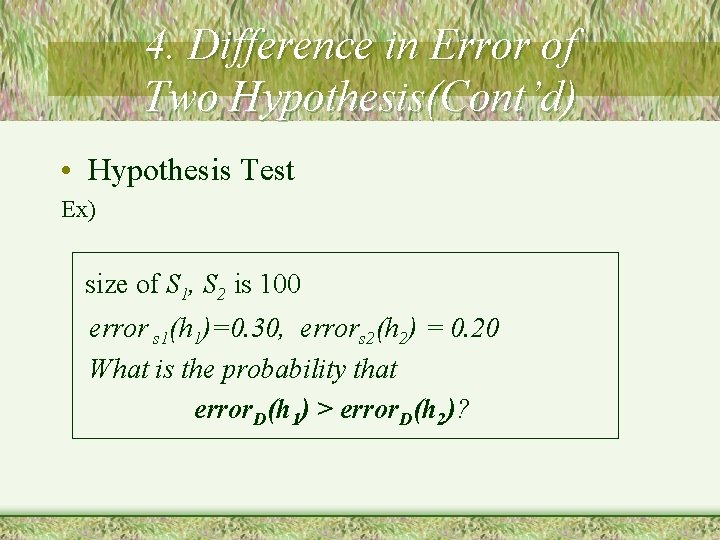4. Difference in Error of Two Hypothesis(Cont’d) • Hypothesis Test Ex) size of S 1, S 2 is 100 error s 1(h 1)=0. 30, errors 2(h 2) = 0. 20 What is the probability that error. D(h 1) > error. D(h 2)?4. Difference in Error of Two Hypothesis(Cont’d) • Solution 1. The problem is equivalent to getting the probability of the following 2. From former expression, 3. Table of Normal distribution shows that associated confidence level for two-sided interval is 90%, so for one-sided interval, it is 95%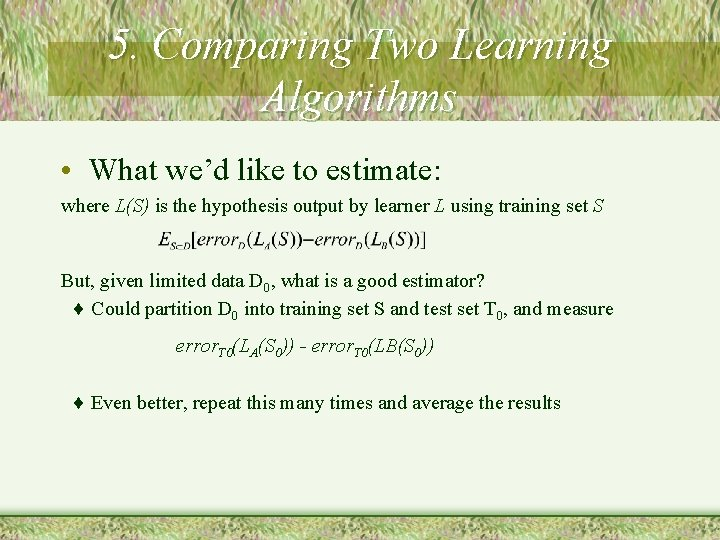5. Comparing Two Learning Algorithms • What we’d like to estimate: where L(S) is the hypothesis output by learner L using training set S But, given limited data D 0, what is a good estimator? Could partition D 0 into training set S and test set T 0, and measure error. T 0(LA(S 0)) - error. T 0(LB(S 0)) Even better, repeat this many times and average the results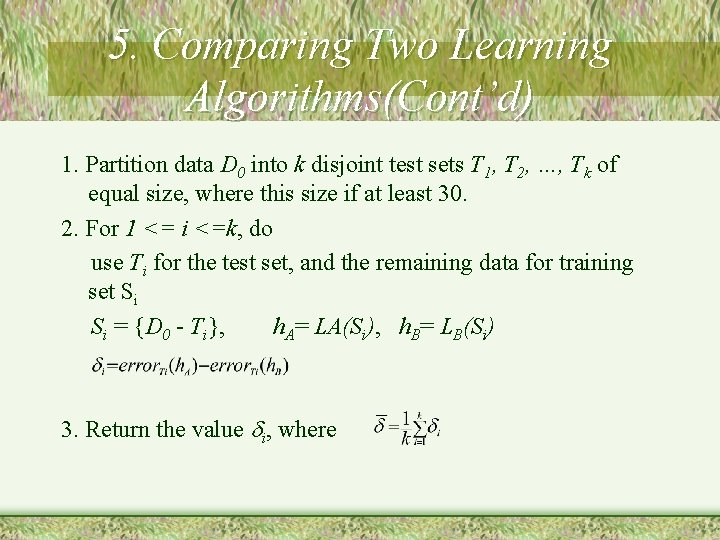5. Comparing Two Learning Algorithms(Cont’d) 1. Partition data D 0 into k disjoint test sets T 1, T 2, …, Tk of equal size, where this size if at least 30. 2. For 1 <= i <=k, do use Ti for the test set, and the remaining data for training set Si Si = {D 0 - Ti}, h. A= LA(Si), h. B= LB(Si) 3. Return the value i, where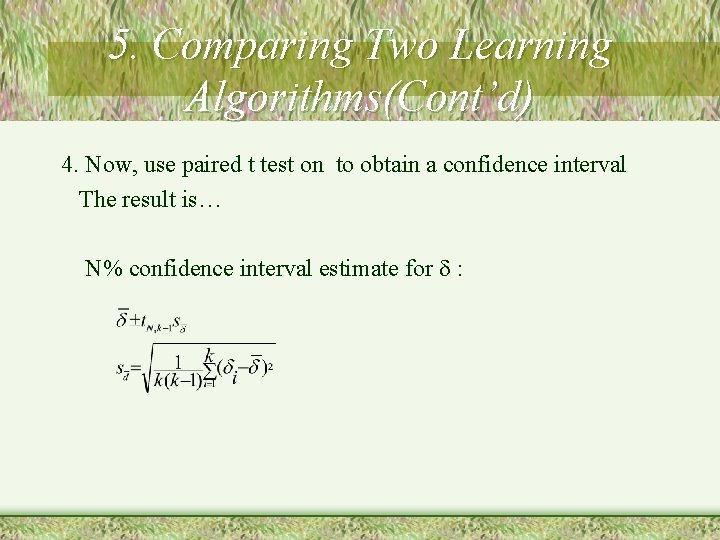5. Comparing Two Learning Algorithms(Cont’d) 4. Now, use paired t test on to obtain a confidence interval The result is… N% confidence interval estimate for :